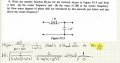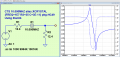# Value of transfer function at the corner freq.

#### guyvsdcsniper

Joined Sep 19, 2023
9
I am trying to solve part b of this problem.

I first found the transfer function, which is the impedance of the vertical branch divided by the series sum of the impedances.

After, I took the limit of the transfer function of this LC low pass filter at low and high ##/omega##.

I then found the corner frequency by setting ##H_(low) and ##H_(high)## equal to each other.

Next, I take the magnitude of the transfer function, but the expression I get for it causes the denominator be zero, which is bad. Ideally, at the corner frequency, I should get ##\frac{1}{sqrt(2)}###### Papabravo

Joined Feb 24, 2006
20,600
Your algebra is faulty. Go back and do it again with a bit more care.

#### guyvsdcsniper

Joined Sep 19, 2023
9
Your algebra is faulty. Go back and do it again with a bit more care.
Im still stumped on where im going wrong. I've redone it about 3 times and still come out with the wrong answer.

It has to be a problem in the denominator but Im not seeing it.

#### guyvsdcsniper

Joined Sep 19, 2023
9
Actually, the corner frequency I found is just the corner freq for this filter. So all I need to do is plug in the values and divide the corner freq by 2*pi and that has to be the value of H at the corner freq, right?

#### Papabravo

Joined Feb 24, 2006
20,600
A resonant circuit does not have a corner frequency. It does have a point, at resonance, where the total impedance approaches zero. Real circuits will actually have a non-zero impedance at this point since ideal components do not exist. If you do an AC analysis, as you would do for a crystal for example you would see a pair of peaks at the series resonant and parallel resonant points. The magnitude of the peak will depend on the values of the non-reactive components.Last edited:

#### guyvsdcsniper

Joined Sep 19, 2023
9
A resonant circuit does not have a corner frequency. It does have a point, at resonance, where the total impedance approaches zero. Real circuits will actual have a non-zero impedance at this point since ideal components do not exist.
Then why does my book say to list the corner frequency?

#### guyvsdcsniper

Joined Sep 19, 2023
9
A resonant circuit does not have a corner frequency. It does have a point, at resonance, where the total impedance approaches zero. Real circuits will actually have a non-zero impedance at this point since ideal components do not exist. If you do an AC analysis, as you would do for a crystal for example you would see a pair of peaks at the series resonant and parallel resonant points. The magnitude of the peak will depend on the values of the non-reactive components.

View attachment 303189
So what does this mean for me when solving part b of my question? Is this why I am getting a 1/0?

#### guyvsdcsniper

Joined Sep 19, 2023
9
Here is a simulation of your example showing the magnitude (35dB) and the approximate resonant frequency of approximately 159 Hz.

View attachment 303190
OK I did get 159 hz for what I was calling the corner frequency, which is reassuring. What is this program called?

#### Papabravo

Joined Feb 24, 2006
20,600
You are looking at a rational function with a denominator very close to zero IF you have IDEAL components. I have no clue what your book is or is not telling you. In order to evaluate such a function there must be some additional assumptions. For example the series resistance of the inductor or the equivalent series resistance of the capacitor. Simulators make such assumptions to avoid having to solve a singular matrix. My initial mistake was thinking (for a moment) that you had to evaluate a finite 1st order transfer function. I apologize for that. It is a legitimate 2nd order system and that is why you have a resonance which is a very different animal from a corner frequency.

#### Papabravo

Joined Feb 24, 2006
20,600
OK I did get 159 hz for what I was calling the corner frequency, which is reassuring. What is this program called?
This simulator is called LTspice and it is a free download from Analog Devices.
https://www.analog.com/en/design-center/design-tools-and-calculators/ltspice-simulator.html

You can get actual values for the magnitude response by taking frequencies close to but not exactly equal to the computed resonant frequency. For example, try 155 Hz. or 162 Hz.

ETA: The simulation command is doing a frequency sweep with a 1 VAC source using with 96 points per decade from 1 Hz to 1 kHz. I chose those numbers because I knew where the peak would be. 35 dB on a 1 VAC source would be about 56 VAC peak

Last edited:

#### guyvsdcsniper

Joined Sep 19, 2023
9
You are looking at a rational function with a denominator very close to zero IF you have IDEAL components. I have no clue what your book is or is not telling you. In order to evaluate such a function there must be some additional assumptions. For example the series resistance of the inductor or the equivalent series resistance of the capacitor. Simulators make such assumptions to avoid having to solve a singular matrix. My initial mistake was thinking (for a moment) that you had to evaluate a finite 1st order transfer function. I apologize for that. It is a legitimate 2nd order system and that is why you have a resonance which is a very different animal from a corner frequency.
Ok thanks that makes sense.

How would I approach find the degrees of phase shift below and above the resonant freq? I see graphically that is 180 degrees, I just dont know how to calculate it or what formula to use?

#### Papabravo

Joined Feb 24, 2006
20,600
Ok thanks that makes sense.

How would I approach find the degrees of phase shift below and above the resonant freq? I see graphically that is 180 degrees, I just dont know how to calculate it or what formula to use?
Think about what is going on here. You have a circuit impedance with a very small real part. In the vicinity of resonance, you also have an impedance with a very small imaginary part. The arc tangent of the ratio of small numbers is going to be either 0° or 180°. The real and imaginary parts of the LC circuit are going to have a hard time producing other phase shifts.

For a normal transfer function at a point other than resonance, the phase is the arc tangent of the imaginary part over the real part. REMEMBER the imaginary part does not include the imaginary unit "j".